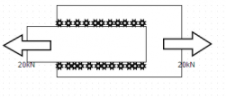Courses

# Test: Butt Joints & Fillet Joints

## 10 Questions MCQ Test Machine Design | Test: Butt Joints & Fillet Joints

Description
This mock test of Test: Butt Joints & Fillet Joints for Mechanical Engineering helps you for every Mechanical Engineering entrance exam. This contains 10 Multiple Choice Questions for Mechanical Engineering Test: Butt Joints & Fillet Joints (mcq) to study with solutions a complete question bank. The solved questions answers in this Test: Butt Joints & Fillet Joints quiz give you a good mix of easy questions and tough questions. Mechanical Engineering students definitely take this Test: Butt Joints & Fillet Joints exercise for a better result in the exam. You can find other Test: Butt Joints & Fillet Joints extra questions, long questions & short questions for Mechanical Engineering on EduRev as well by searching above.
QUESTION: 1

### If force act in a direction parallel to the direction of weld, then fillet weld is called as?

Solution:

Explanation: Parallel fillet weld consist of force acting in a direction of weld.

QUESTION: 2

### Convex weld is generally preferred over normal weld.

Solution:

Explanation: There is more stress concentration in convex weld and hence is less preferred.

QUESTION: 3

### Parallel fillet weld and transverse fillet weld both have the plane in which maximum shear stress occurs at 45’ to the leg dimension.

Solution:

Explanation: Transverse fillet weld has that plane inclined at 67.5 .

QUESTION: 4

The length of each of the two equal sides of a parallel fillet weld is called

Solution:

Explanation: Leg is the length of each of the two equal sides of a parallel fillet weld.

QUESTION: 5

Relation between throat and leg for a parallel fillet weld is

Solution:

Explanation: Throat is the minimum cross section of the weld and that plane lies at 45’ for a parallel fillet weld.

QUESTION: 6

If length of weld is l and leg h, then area of throat can be given by

Solution:

Explanation: Area=t x l where t=h Cos (45’).

QUESTION: 7

A steel plate is welded to another steel plate as shown in the figure. This is an example of transverse fillet weld.Solution:

Explanation: The force acts parallel to the direction of weld and hence is an example of double parallel fillet weld.

QUESTION: 8

Referring to figure , determine the required length of the weld joining two steel plates which are 10mm wide and 10mm thick. The permissible shear stress in the weld is 80N/mm²Solution:

Explanation: P=2 x 0.707 hlτ or 20000=1.414x10xlx80 or l=17.68mm.

QUESTION: 9

The two plates are welded as shown in the figure . It is an example ofSolution:

Explanation: In two welds force acts parallel as well as perpendicular to the direction of weld.

QUESTION: 10

With reference to figure , a plate, 75mm wide and 10mm thick, is joined with another plate as shown. The permissible tensile and shear stress in the welds are 50/mm² and 40N/mm². Determine the required length of each parallel fillet weld if length of transverse weld is 40mm.Solution:

Explanation: 0.707hl₁σ+1.414hl₂τ=20000 or 0.707x10x50x40+1.414x10xl₂x40=20000.